# Introduction to Dynamic Ranges

An introduction to Dynamic Ranges

The VLOOKUP function is often used to find information that is stored within tables in Excel. So for example if we have a list of people’s names and ages: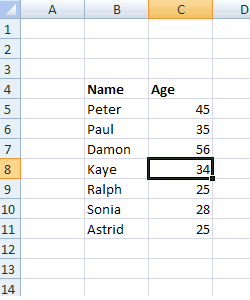And then we can in a nearby cell use the function VLOOKUP to determine Paul’s age: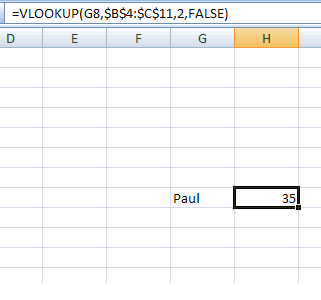So far this is a fairly standard. But what happens if we need to add some more names to the list ? The obvious thought would be to modify the range in the VLOOKUP. However, in a really complex model, there may be several references to the VLOOKUP. This means that we would have to change each reference – assuming that we knew where they were.

However Excel provides an alternative way – called a DYNAMIC range. This is a range that expands an updates automatically. This is perfect if your lists are for ever expanding (e.g month on month sales data).

To set up a dynamic range we need to have a range name – so we’ll call ours AGE_DATA. The approach for setting up dynamic ranges differs between Excel 2007 and earlier versions of Excel:

In Excel 2007, click on “Define Name” under formulae:

In earlier versions of Excel click on “Insert” and then Names” .

In the pop up box, enter the name of our dynamic range – which is “AGE DATA”: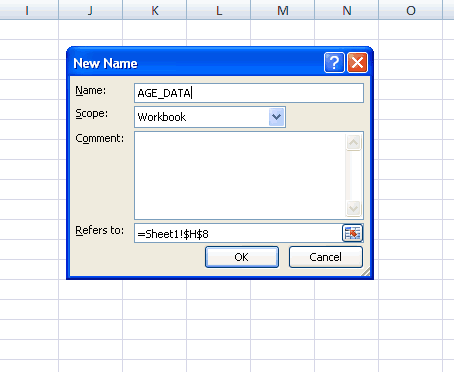In the box labeled “Refers To” we need to enter the range of our data. This is will be an achieved used by an OFFSET function. This has 5 arguments:

=OFFSET(Reference, Rows, Cols, Height, Width)

– The Reference is the address of the TOP LEFT corner of our range – in this case cell B5
– The Rows is the number of rows from the TOP LEFT that we want that range to be – which will be 0 in this case
– The Cols is the number of rows from the TOP LEFT that we want that range to be – which will be 0 in this case
– The Height of the range – see below for this
– The Width of the range – this is 2 has we have TWO columns in our range (the persons name and their age)

Now the height of the range will have to vary depending on the number of entries in our table (which is currently 7).

Of course we want a way of counting up the rows in our table that updates automatically – so one way of doing this is to use the COUNTA function. This just counts up the number of non blank cells in a range. As our names are in column B, the number of entries in our data is COUNTA(B:B).

Note that if you were to put this in a cell you would get the value 8 – as it includes the header Names. However, that it is immaterial.
So in the “Refers To” box we put:

=OFFSET(\$B\$5,0,0,counta(B:B),2)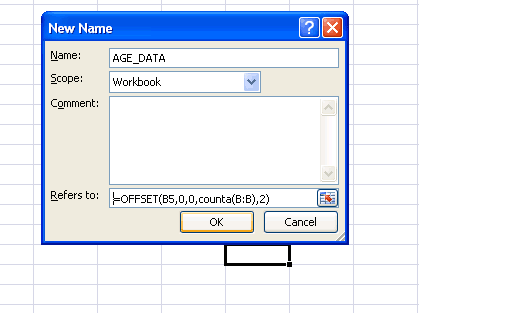And click the OK button. Our dynamic range is now created.
Now return to the VLOOKUP formulae and replace the range \$B:4:\$C11 with the name of our new dynamic range AGE_DATA so we have: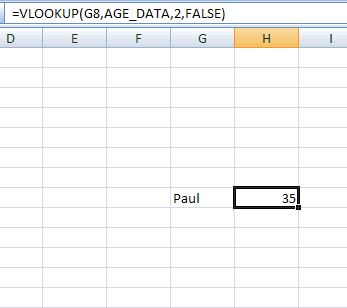So far nothing has changed. However if we add a few more names to our table: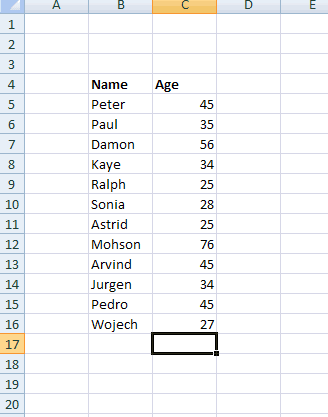And in the cell where we had Paul, replace it with a new name such as Pedro (that wasn’t on original list):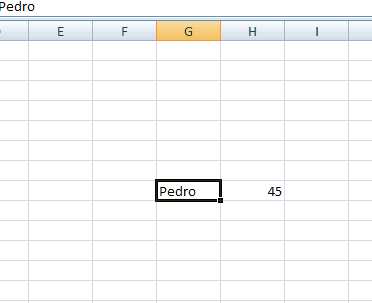And we see that Excel has automatically returned Pedro’s age – even though we haven’t changed the VLOOKUP formulae. Instead the scope of the dynamic range has increased to include the extra names.
Dynamic ranges are very useful when we have increasing volumes of data – especially when VLOOKUP and PIVOT tables are required.